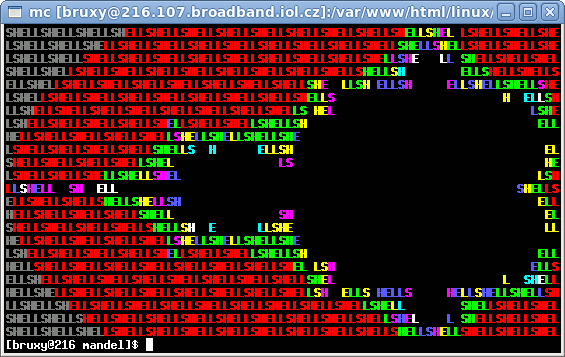Published: 4. 3. 2010   Category: GNU/Linux

# Mandelbrot set computed in BASH

The following 512 bytes shell script counts and display Mandelbrot set on standard Linux/ANSI terminal.

Copy and paste following code to the text console:

``` #!/bin/bash S0=S;S1=H;S2=E;S3=L;S4=L;e=echo;b=bc;I=-1;for x in {1..24}; do R=-2;for y in {1..80};do B=0;r=0;i=0;while [ \$B -le 32 ];do r2=`\$e "\$r*\$r"|\$b`;i2=`\$e "\$i*\$i"|\$b`;i=`\$e "2*\$i*\$r+\$I"|\$b`; r=`\$e "\$r2-\$i2+\$R"|\$b`;: \$((B+=1));V=`\$e "(\$r2 +\$i2)>4"|\$b`; if [ "\$V" -eq 1 ];then break;fi;done; if [ \$B -ge 32 ];then \$e -n " ";else U=\$(((B*4)/15+30));\$e -en "\E[01;\$U""m";C=\$((C%5)); eval "\$e -ne \\$E\\$S\$C";: \$((C+=1));fi;R=`\$e "\$R+0.03125"|\$b` done;\$e -e "\E[m\E(\r";I=`\$e "\$I+0.08333"|\$b`;done #(c)BruXy ```The computing is quite slow, because there is bc called for floating point operations.

If you do not want spent a lot of machine time try this command:

``` exec 3<>/dev/tcp/bruxy.regnet.cz/80 echo -e "GET http://bruxy.regnet.cz/linux/mandel/mandel.ansi HTTP/1.0\n" >&3 cat <&3 ```

This code (in GNU/Linux) opens TCP connection to this web site on port 80 (HTTP) with the file descriptor number 3. Then HTTP command GET is send to the address and the port and output of 3 is displayed on the standard output with cat.

## New performance improved version

``` S0=S;S1=H;S2=E;S3=L;S4=L;e=echo;b=bc;I=-1;t=/tmp/m\$\$;for x in {1..13}; do R=-2;for y in {1..80};do B=0;r=0;i=0;V=0;while [ \$B -le 32 -a \$V -eq 0 ]; do read rt i V<<<`\$b<<<"r2=\$r^2;i2=\$i^2;r2-i2+(\$R);2*(\$r*\$i)+(\$I);(r2+i2)>4"`; r=\$rt;: \$((B++));done;if [ \$B -ge 32 ];then \$e -n " "; else \$e -en "\E[01;\$(((B*4)/15+30))""m"; eval "\$e -ne \\$E\\$S\$((C++%5))"; fi; R=`\$b<<<"\$R+0.03125"`;done;\$e -e "\E[m\E(\r";I=`\$b<<<"\$I+0.08333"`; done|tee \$t;head -n 12 \$t|tac #(c)BruXy ```

This new code obfuscates two speed improvements. The first one is a lower number of calling bc. There are 3 calls instead of 7. Because four calls were made in the inner loop and they were reduced into only one call the speed increases rapidly. The second improvement consist in a fact, that computed part of the Mandelbrot set is symmetric. So I compute only first half of the set, data are written into a file. Then they are „mirrored“ on a screen with command tac (line reversed cat).

Total running time of script fell from 4:20 to only 0:39 seconds on my computer.

## Fixed point version

``` #!/bin/bash p=\>\>14 e=echo\ -ne\ S=(S H E L L) I=-16384 t=/tmp/m\$\$; for x in {1..13}; do \ R=-32768; for y in {1..80}; do B=0 r=0 s=0 j=0 i=0; while [ \$((B++)) -lt 32 -a \ \$((\$s*\$j)) -le 1073741824 ];do s=\$((\$r*\$r\$p)) j=\$((\$i*\$i\$p)) t=\$((\$s-\$j+\$R)); i=\$((((\$r*\$i)\$p-1)+\$I)) r=\$t;done;if [ \$B -ge 32 ];then \$e\ ;else #---::BruXy::- \$e"\E[01;\$(((B+3)%8+30))m\${S[\$((C++%5))]}"; fi;R=\$((R+512));done;#----:::(c):::- \$e "\E[m\E(\r\n";I=\$((I+1311)); done|tee \$t;head -n 12 \$t| tac #-----:2 O 1 O:- ```

This last version beats the previous one completely. Main improvement I made is rewriting computational part of code to fixed point arithmetic. The real time of this solution is only 1.165 seconds on my box! There are also some more changes in BASH code that makes source slightly reduced for few bytes and also make it more cryptic. I changed the routine for ANSI color mapping so the output colors are more psychedelic :-)

```SHELLSHELLSHELLSHELLSHELLSHELLSHELLSHELLSHELLSHELLSHELLSHELLSHEL LSHELLSHELLSHEL
LSHELLSHELLSHELLSHELLSHELLSHELLSHELLSHELLSHELLSHELLSHELLSHELLSHELLSHELLSHELLSHEL
LSHELLSHELLSHELLSHELLSHELLSHELLSHELLSHELLSHELLSHELLSHELLSHE   LLSHELLSHELLSHELLS
HELLSHELLSHELLSHELLSHELLSHELLSHELLSHELLSHELLSHELLSHELLSH         ELLSHELLSHELLSH
ELLSHELLSHELLSHELLSHELLSHELLSHELLSHELLSHELLSHELLSHELLSHELL     SHELLSHELLSHELLSH
ELLSHELLSHELLSHELLSHELLSHELLSHELLSHELLSHELLSHE    L                  LSHEL LSHEL
LSHELLSHELLSHELLSHELLSHELLSHELLSHELLSHELLSHELLSH                           ELLSH
ELLSHELLSHELLSHELLSHELLSHELLSHELLSHELLSHELL                                SHELL
SHELLSHELLSHELLSHELLSHELLSHELLSHELLSHELLSHE                                  L L
SHELLSHELLSHELLSHELLSHELLSH  ELL SHELLSHE                                   LLSH
ELLSHELLSHELLSHELLSHELLSHEL           LSH                                    ELL
SHELLSHELLSHELLSHELLSHEL                L                                   SHEL
LSHELLSHELLSHELLSHEL                                                       LSHEL
SHELLSHELLSHELLSHELLSHEL                L                                   SHEL
ELLSHELLSHELLSHELLSHELLSHEL           LSH                                    ELL
SHELLSHELLSHELLSHELLSHELLSH  ELL SHELLSHE                                   LLSH
SHELLSHELLSHELLSHELLSHELLSHELLSHELLSHELLSHE                                  L L
ELLSHELLSHELLSHELLSHELLSHELLSHELLSHELLSHELL                                SHELL
LSHELLSHELLSHELLSHELLSHELLSHELLSHELLSHELLSHELLSH                           ELLSH
ELLSHELLSHELLSHELLSHELLSHELLSHELLSHELLSHELLSHE    L                  LSHEL LSHEL
ELLSHELLSHELLSHELLSHELLSHELLSHELLSHELLSHELLSHELLSHELLSHELL     SHELLSHELLSHELLSH
HELLSHELLSHELLSHELLSHELLSHELLSHELLSHELLSHELLSHELLSHELLSH         ELLSHELLSHELLSH
LSHELLSHELLSHELLSHELLSHELLSHELLSHELLSHELLSHELLSHELLSHELLSHE   LLSHELLSHELLSHELLS
LSHELLSHELLSHELLSHELLSHELLSHELLSHELLSHELLSHELLSHELLSHELLSHELLSHELLSHELLSHELLSHEL
SHELLSHELLSHELLSHELLSHELLSHELLSHELLSHELLSHELLSHELLSHELLSHELLSHEL LSHELLSHELLSHEL
```